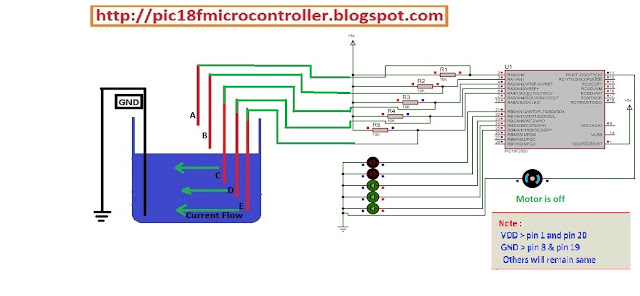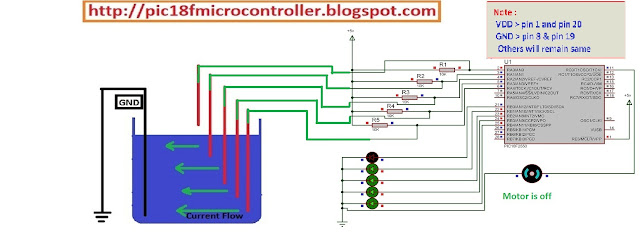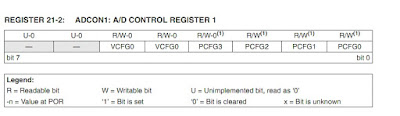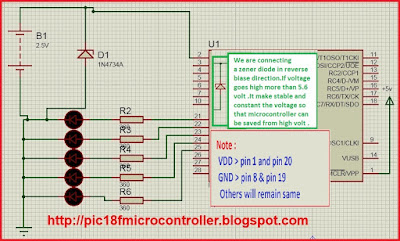# Learn Embedded Programming [Step by Step]

## Water Level Indicator Project using Microcontroller

Water tank controller  is an embedded project where motor switch automatically controlled by a microcontroller .When tank's water level is very low , the microcontroller system turns on the motor switch , Until the water level is full or 100% and the motor switch remains on . When microcontroller get notification tank is full , it turns off the switch of motor . Until the tank is empty this stage remains on .
Now the question is , how can we get notification about water level ? We will apply a technique . Look at the picture given below .Microcontroller Project : Water Tank Controller using pic18f2550 Microcontroller

Here microcontroller pins (RA0-RA4)  are connected to VDD through five 10k Ohm resistors . Five cables are connected to the points between resistors first end points and mcu (RA0-RA4)pin points . Those five (A,B,C,D,E) cables are hanged  into the tank at different five levels(A,B,C,D,E) so that each cable represents each of five levels individually .Microcontroller Project : Water Tank Controller using pic18f2550 Microcontroller
Once water level goes to E level , it makes enable the connection between GND and 'E' level cable . So , it makes the RA4 pin GND(logical 0) and we get notification the tank is empty . Consequently the system turns on the motor of switch .Water Tank Controller Project using Microcontroller

When it's full, all the mcu pins(RA0-RA4) goes to low or 0 . We get the notification tank is full the motor needs to be turned off . That's the working process of this system.

### Souece Code :

``````

void main() {
ADCON1=0x0F;                 // Configure RA) pin as input
CMCON=7;
TRISC.F0=0;
TRISA.F0=1;
TRISA.F1=1;
TRISA.F2=1;
TRISA.F3=1;
TRISA.F4=1;
TRISB=0x00;
while(1){
if(PORTA.F4==0 && PORTA.F3==0 && PORTA.F2==0 &&  PORTA.F1==0 && PORTA.F0==0){
PORTB.F0=1;
PORTB.F1=1;                                         // 100% full
PORTB.F2=1;
PORTB.F3=1;
PORTB.F4=1;
PORTC.F0=0;   //motor turned off
}
if(PORTA.F4==0 && PORTA.F3==0 && PORTA.F2==0 &&  PORTA.F1==0 && PORTA.F0==1){
PORTB.F0=0;
PORTB.F1=1;                                        // 80% full
PORTB.F2=1;
PORTB.F3=1;
PORTB.F4=1;}
if(PORTA.F4==0 && PORTA.F3==0 && PORTA.F2==0 &&  PORTA.F1==1 && PORTA.F0==1){
PORTB.F0=0;
PORTB.F1=0;
PORTB.F2=1;                                            // 60% full
PORTB.F3=1;
PORTB.F4=1;

}
if(PORTA.F4==0 && PORTA.F3==0 && PORTA.F2==1 &&  PORTA.F1==1 && PORTA.F0==1){
PORTB.F0=0;
PORTB.F1=0;                                            // 40% full
PORTB.F2=0;
PORTB.F3=1;
PORTB.F4=1;
}
if(PORTA.F4==0 && PORTA.F3==1 && PORTA.F2==1 &&  PORTA.F1==1 && PORTA.F0==1){
PORTB.F0=0;
PORTB.F1=0;
PORTB.F2=0;
PORTB.F3=0;                                              // 20% full
PORTB.F4=1;
PORTC.F0=1;   //motor turned on
}
}
}``````

### Circuit :Water Tank Controller Project using MicrocontrollerWater Tank Controller Project using Microcontroller

## Battery Charge Level Indicator (5volt) using Microcontroller

Battery Charge Level Viewer is a microcontroller based small project which shows charge level of battery using 5 LEDs . Generally battery becomes full at 5volts . Remember that an ADC channel of pic18f2550 can count from 0 to 1023 . Because it's ADC channel register is 10bits. As we like to  measure 5volt  . We will read the value of adc channel and will convert it to voltage . After converting into voltage we get a understandable value and we shows it through 5 green LEDs. It's the basic concept .

### ADC(Analog to Digital Converter ) :

We need a basic knowledge about ADC . Let's  take a look at here :ADCON1 Register

Basically ADC is like as voltage divider . According to voltage It produce output .

bit 5 : VCFG0: Voltage Reference Configuration bit (VREF- source)
1 = VREF- (AN2)
0 = VSS or 0 volt

bit 4 :VCFG0: Voltage Reference Configuration bit (VREF+ source)
1 = VREF+ (AN3)
0 = VDD or 5volt

We will set  VCFG0[bit 5]=0and VCFG0[bit4]=0 . So we will get highest value 5volt and lowest value 0volt.The ADCON1 is a 10 bit register that means  2 to the power 10  is it's highest counting capacity and result is 1024 . So this register can count from 0 to 1023 . When 0 volt , we get reading at RA0 pin  0 .When 5 volt , we get reading at RA0 pin 1023. It means 5volt equivalent to 1023 .

If  1023 reading value    equal   to 5 volt.
So 1      reading value    equal   to  5/1023 volt

## Source Code :

``````

void main() {
int source=0;
ADCON1=0x0E;                 // Configuring RA0 pin as input
CMCON=7;
TRISB=0x00;
PORTB.F0=0;
PORTB.F1=0;
PORTB.F2=0;
PORTB.F3=0;
PORTB.F4=0;

while(1){

PORTB.F0=0;
PORTB.F1=0;
PORTB.F2=0;
PORTB.F3=0;
PORTB.F4=1;
}
PORTB.F0=0;
PORTB.F1=0;
PORTB.F2=0;
PORTB.F3=1;
PORTB.F4=1;
}
PORTB.F0=0;
PORTB.F1=0;
PORTB.F2=1;
PORTB.F3=1;
PORTB.F4=1;
}
PORTB.F0=0;
PORTB.F1=1;
PORTB.F2=1;
PORTB.F3=1;
PORTB.F4=1;
}
PORTB.F0=1;
PORTB.F1=1;
PORTB.F2=1;
PORTB.F3=1;
PORTB.F4=1;
}
}
}``````

## Circuit :circuit

## Digital Voltmeter project Using Microcontroller

Digital Voltmeter measure voltage between two point in circuit . Now we are going to make a digital voltmeter which can measure 50 volt maximum . We will use pic18f2550 microcontroller and it's ADC pin . Remember that an ADC channel of pic18f2550 can count from 0 to 1023 . Because it's ADC channel register is 10bits. As we like to  measure 50 volt , we need to apply a simple technique . So we have to convert 50 volt to 5 volt  and we get reading 1023 at adc channel  for 50 volt .
For converting we will connect 68k ohm, 22k ohm and 10k ohm resistors in serial so that during applying 50 volt it makes 5 volt drop at 10k ohm resistor . It's the main theme .

### ADC(Analog to Digital Converter ) :

We need a basic knowledge about ADC . Let's  take a look at here :ADCON1 Register

Basically ADC is like as voltage divider . According to voltage It produce output .

bit 5 : VCFG0: Voltage Reference Configuration bit (VREF- source)
1 = VREF- (AN2)
0 = VSS or 0 volt

bit 4 :VCFG0: Voltage Reference Configuration bit (VREF+ source)
1 = VREF+ (AN3)
0 = VDD or 5volt

We will set  VCFG0[bit 5]=0and VCFG0[bit4]=0 . So we will get highest value 5volt and lowest value 0volt.The ADCON1 is a 10 bit register that means  2 to the power 10  is it's highest counting capacity and result is 1024 . So this register can count from 0 to 1023 . When 0 volt , we get reading at RA0 pin  0 .When 5 volt , we get reading at RA0 pin 1023. It means 5volt equivalent to 1023 .

If  1023 reading value    equal   to 50 volt.
So 1      reading value    equal   to  50/1023 volt

### Source Code :

``````

sbit LCD_RS at LATB7_bit;
sbit LCD_EN at LATB6_bit;
sbit LCD_D4 at LATB5_bit;
sbit LCD_D5 at LATB4_bit;
sbit LCD_D6 at LATB3_bit;
sbit LCD_D7 at LATB2_bit;

sbit LCD_RS_Direction at TRISB7_bit;
sbit LCD_EN_Direction at TRISB6_bit;
sbit LCD_D4_Direction at TRISB5_bit;
sbit LCD_D5_Direction at TRISB4_bit;
sbit LCD_D6_Direction at TRISB3_bit;
sbit LCD_D7_Direction at TRISB2_bit;
char volt[]="        ";
char mv[]="v";
void main() {
double source=0;
float calv=0;
float sum=0;
float mult=0;
ADCON1=0x0E;                 // Configure RA) pin as input
CMCON=7;
Lcd_Init();                        // Initialize LCD
Lcd_Cmd(_LCD_CLEAR);               // Clear display
Lcd_Cmd(_LCD_CURSOR_OFF);
Lcd_Out(2,15,mv);
while(1){
calv=(source*50.50)/1023;
floattostr(calv,volt);
Lcd_Out(1,1,"Volt Meter");
Lcd_Out(2,1,"V=");
Lcd_Out(2,4,volt);
}
}

``````

## Thank You!

Ain't getting any visitors!

## Featured Post

### Bluetooth Based Temperature Meter Project using Microcontroller and MikroC USART Terminal## Tags

: (1) 18F2550 (1) 36KHz (3) and (1) arduino (1) Based (1) battery (1) Bipolar (1) Blinking (1) blinks (1) Bluetooth (1) button (1) circuit (1) clock (1) control (1) Db9 (1) DC Motor (2) digital (2) display (2) DS1307 (1) electronic (1) flash (1) flashing (1) HC-06 (1) home (1) how (1) How to (10) i2c tutorial (1) in (1) indicator (1) interface (8) interfacing (3) Interrupt (3) Introduction (1) IR Receiver (4) key pad (1) keyboard (1) keypad (1) lavel (1) Lcd 16x2 (2) lcd 2x16 (2) led (1) lm35 (2) LPG (1) machine (1) make (1) making (1) matrix (1) max232 (1) meter (2) microchip (4) microchips (3) mikroC (5) musical (1) NEC Protocol (4) pcb (5) PIC (3) pic controller (11) pic proteus (1) Pic Tutorial (12) pic18 (2) pic18f2550 (11) picRFモジュール (1) proteus (6) push (1) push button (1) PWM (1) real (1) Rs 232 (1) Rs232 (1) scroll (1) scrolling (1) Serial Port (1) simulation (2) step by step (7) step bystep (1) text (2) time (1) timer (4) timer0 (4) tone (1) tutorial (2) Unipolar (1) USB (1) usb 1.0 (1) USB HID (1) using (9) voltmeter (1) voting (1) with (2) work (1)

## Live Traffic Feed

Live Traffic Feed
Visitor Tracking